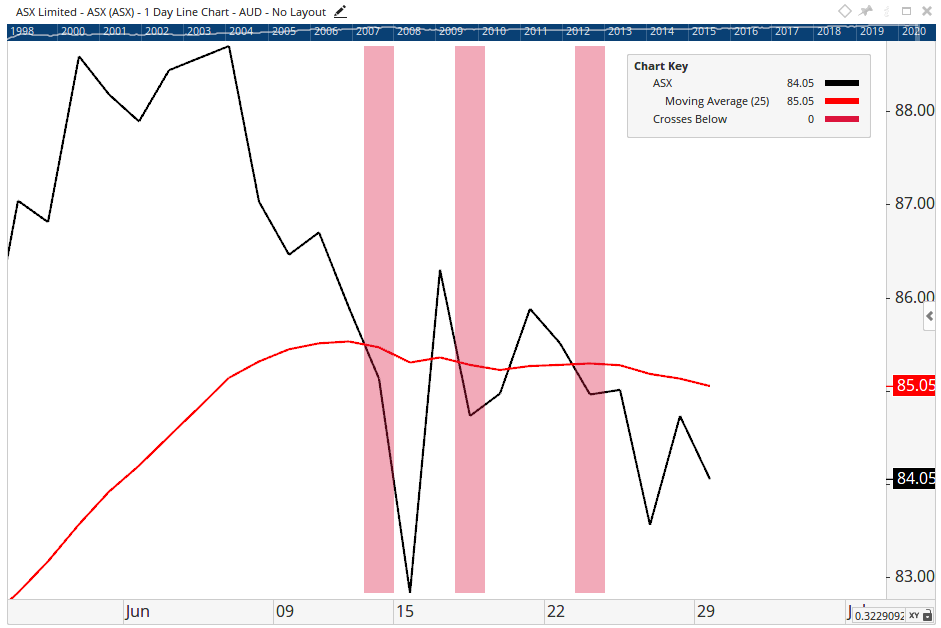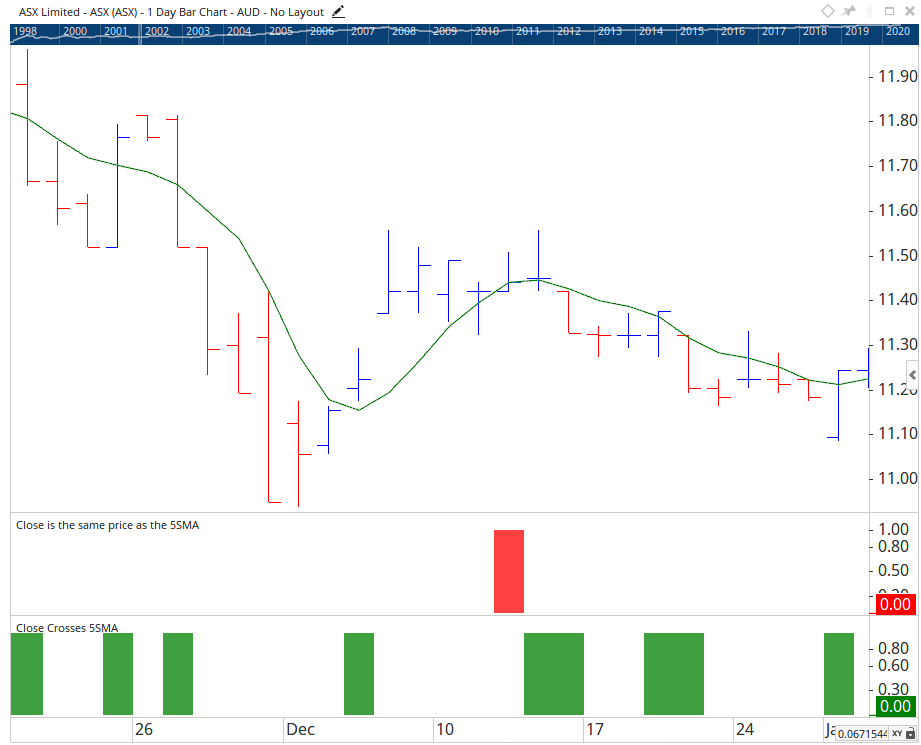# Crosses Operators

Author: Optuma Team Last updated: Jun 30, 2020 14:05

## Overview

Optuma includes 3 Crosses type Operators for use in Scripting:

• CrossesAbove - Looks for instances where Function A crosses above the value of Function B
• CrossesBelow - Looks for instances where Function A crosses below the value of Function B
• Crosses - Looks for instances where Function A crosses above OR below the value of Function B

This is different to the Greater Than > and Less Than < Operators, as the Crosses operators will only show the first instance the values cross and won’t trigger again until the value has crossed above / below again.

## Examples

Closing Price Crosses Above 25EMA

**CLOSE() CrossesAbove MA(BARS=25, STYLE=Exponential)Closing Price Crosses Below 25EMA

**CLOSE() CrossesBelow MA(BARS=25, STYLE=Exponential)## Optuma v1.6 Update

With the release of the Optuma v1.6 update the Crosses, CrossesAbove and CrossesBelow operators have been adjusted so that values equal to each other do not count as a cross.

### Example

Close Crossing 5SMA in v1.5 vs v1.6In Optuma v1.5 and earlier you can see in the instances where the Moving Average value and the Closing value matched, the script showed a true result.In Optuma v1.6 the equal values no longer trigger a true result.
</span>